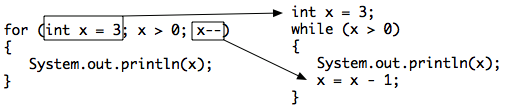# 7.3. For Loops¶

A for loop is usually used when you know how many times you want the loop to execute. A for loop has 3 parts: initialization, condition, and change. The parts are separated by semicolons (`;`).

Note

Each of the three parts of a `for` loop declaration is optional (initialization, condition, and change), but the semicolons are not optional.

```for (initialization; condition; change)
```

One of the strange things about a `for` loop is that the code doesn’t actually execute where you see it in the declaration. The code in the initialization area is executed only one time before the loop begins, the condition is checked each time through the loop and the loop continues as long as the condition is true, at the end of each execution of the body of the loop the changes are done. When the loop condition is false execution will continue at the next statement after the body of the loop.

You can compare a `while` loop to a `for` loop to understand that a `for` loop actually executes like a `while` loop does if you use the `while` loop to repeat the body of the loop a specific number of times.Figure 1: Showing how a for loop maps to a while loop

6-3-1: What do you think will happen when you run the code below? How would it change if you changed line 11 to <code>i = 3</code>?

The method printPopSong prints the words to a song. It initializes the value of the variable i equal to 5 and then checks if i is greater than 0. Since 5 is greater than 0, the body of the loop executes. Before the condition is checked again, i is decreased by 1. When the value in i is equal to 0 the loop stops executing.

Note

The number of times a loop executes can be calculated by (largestValue - smallestValue + 1). By the largest value I mean the largest value that allows the loop to execute and by the smallest value I mean the smallest value that allows the loop to execute. So in the code above the largest value is 5 and the smallest value that allows the loop to execute is 1 so this loop executes (5 - 1 + 1 = 5 times).

How many times does the code above print the lines to the song?

Note

You can also calculate the number of times a loop executes as the value that ends the loop minus the starting value. In this case the loop ends when i is 3 so (3 - 0 = 3).

6-3-2: What does the following code print?

```for (int i = 3; i < 8; i++)
{
System.out.print(i + " ");
}
```
• 3 4 5 6 7 8
• This loop starts with i equal to 3 but ends when i is equal to 8.
• 0 1 2 3 4 5 6 7 8
• What is i set to in the initialization area?
• 8 8 8 8 8
• This would be true if the for loop was missing the change part `(int i = 3; i < 8; )` but it does increment i in the change part `(int i = 3; i < 8; i++)`.
• 3 4 5 6 7
• The value of i is set to 3 before the loop executes and the loop stops when i is equal to 8. So the last time through the loop i is equal to 7.

6-3-3: What does the following code print?

```for (int i = 1; i <= 10; i++)
{
System.out.print(i + " ");
}
```
• 3 4 5 6 7 8
• What is i set to in the initialization area?
• 0 1 2 3 4 5 6 7 8 9
• What is i set to in the initialization area?
• 1 2 3 4 5 6 7 8 9 10
• The value of i starts at 1 and this loop will execute until i equals 11. The last time through the loop the value of i is 10.
• 1 3 5 7 9
• This loop changes i by 1 each time in the change area.

6-3-4: How many times does the following method print a `*`?

```for (int i = 3; i <= 9; i++)
{
System.out.print("*");
}
```
• 10
• This would be true if i started at 0 and ended at 9. Does it?
• 6
• Since i starts at 3 and the last time through the loop it is 9 the loop executes 7 times (9 - 3 + 1 = 7)
• 7
• How many numbers are between 3 and 9 (including 3 and 9)?
• 9
• This would be true if i started at 0 and the value of i the last time through the loop it was 8.

Mixed up programs